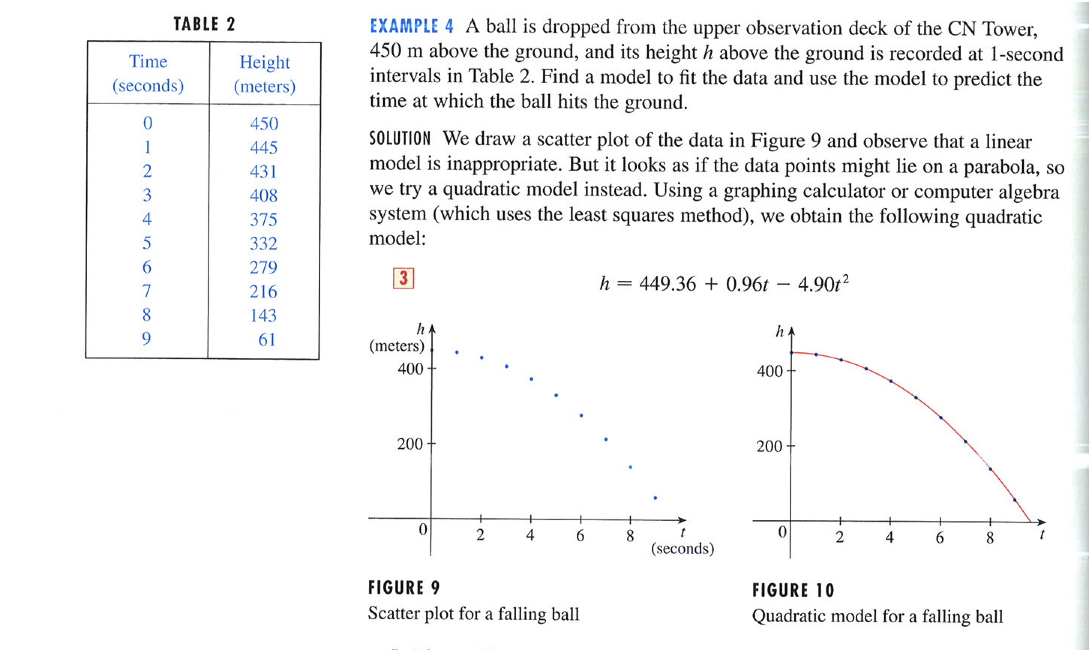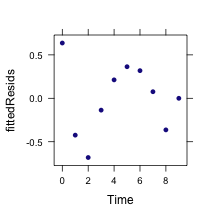A problem from Stewart Calculus: Concepts and Contexts 2/e p. 30.### Questions

• Is the formula stated in the problem the same as one gets by fitting the data in the table?
• According to the formula, what is the ball velocity at $$t=0$$.
• Fit the quadratic model and find the time when the height is zero.
• How would air resistance be expected to change things? (Hint: terminal velocity)
Ball = fetchData("PREP-Toronto-ball.csv")
modHeight = fitModel(Height ~ a * Time^2 + b * Time + c, data = Ball)
findZeros(modHeight(t) ~ t, t.lim = c(5, 15))

##  9.674


An a priori model:

apriori = makeFun(450 - 0.5 * 9.806 * Time^2 ~ Time)


How good are the models?

aprioriResids = with(data = Ball, Height - apriori(Time))
fittedResids = with(data = Ball, Height - modHeight(Time))
plotPoints(fittedResids ~ Time, data = Ball)### Questions:

• Why might the first residual be expected to be larger than the others? (Hint: How do you synchronize the measurement time with the release time?)
• What pattern in the a priori model suggests that the initial velocity was not zero?
• Are the residuals big or small for real data? How would you decide?

Do some research on “terminal velocity” to figure out what would be an appropriate terminal velocity for a ball. One equation you may find is $V_t = \sqrt{\frac{2 m g}{\rho A C_d}},$ where $$m$$ is the mass of the ball, $$g$$ the acceleration due to gravity, $$\rho$$ the density of air, $$A$$ the cross-sectional area of the ball, and $$C_d$$ the “drag coefficient.” You should be able to find values for each of these and a plausible range of values for $$m$$ and $$A$$ for a “ball.” For a sphere, the drag coefficient is $$C_d = 0.47$$.

• Is there any time where, according to the data or the model formula, the velocity is greater than terminal velocity?

### Going further

Build a differential equation with a reasonable model of air drag. In particular, arrange it to reach a plausible terminal velocity. How far is height versus time from a parabola?

Here's a video of a falling ball. Perhaps you can find other data for longer falls. Try to find some compelling data and see if they display terminal velocity.